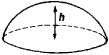Spherical Segment of One Base

Spherical Segment of One Base

a part of a sphere cut off by a plane (see Figure 1). The volume of such a solid is VFigure 1

= πh2(3R - h)/3, and the area of the solid’s curved surface, which is called a zone of one base, is S = 2πRh; here, R is the radius of the sphere, and h is the altitude of the segment.

Site: Follow: Share:
Open / Close